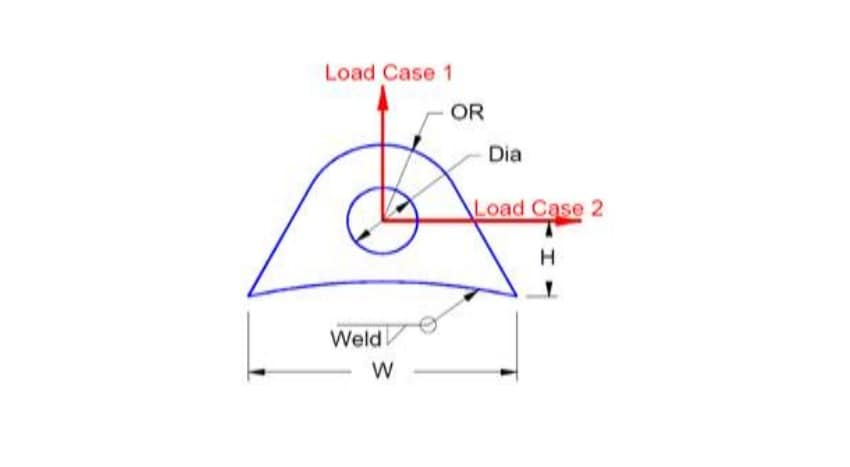LIFTING LUGSLoad = vessel weight empty in lb
W = width in inches
Thick = lug thickness in inches
H = hole height in inches
Dia = hole diameter in inches
OR = outside radius in inches
Weld = leg size
STRESS LIMITS
SA = Allowed stress in tension in psi (SA value can be taken from the material properties of lug material)
SPb = Pin bearing stress in psi 1.6 * SA (1.6 times of allowed stress)
SB = Maximum bending stress in psi 1.5 * SA (1.5 times of allowed stress)
SS = maximum shear stress in psi 0.8 * SA (0.8 times of allowed stress)
SSw = maximum weld shear stress in psi 0.49 * SA (0.49 times of allowed stress)
CALCULATION OF STRESSES FOR THE CORRESPONDING LOADS ACTING ON LIFTING LUGS
The stress values of lifting lugs for the corresponding loads acting can be calculated from the formulae shown below. The calculated stress values should be less than to the stress limits.
Tensile stress ( case 1)
Tensle stress = Load / Area (psi)
Where,
Area = thick* (OR – (Dia/2)) (in2)
The calculated tensile stress value should be less than to allowed stress (SA), otherwise change the dimensions of lifting lug and then proceed again.
PinBearing stress ( case 1 & 2)
Pin stress = Load / Area (psi)
Where,
Area = Dia * Thick (in2)
The calculated pin bearing stress value should be less than to 1.6 times of allowed stress (SPb), otherwise change the dimensions of lifting lug and then proceed again.
Bending stress ( case 2)
Bending stress = (moment * C)/ I (psi)
Where,
Moment = Load * H (in-lb)
Moment of inertia ` = Thick * W^3 / 12 (in4)
C = W/2 (in)
The calculated bending stress value should be less than to 1.5 times of allowed stress (SB), otherwise change the dimensions of lifting lug and then proceed again.
Shear stress (Case 2)
Shear stress = Load / Shear Area (psi)
Where,
Shear area = W * Thick (in2)
The calculated Shear stress value should be less than to 0.8 times of allowed stress (SS) otherwise change the dimensions of lifting lug and then proceed again.
Weld stress (Case 1)
Weld stress = Load / Weld Area (psi)
Where,
Weld area = circumference * Weld (in2)
Circumference = W *2 +Thick*2+Weld*4 (in)
The calculated Weld stress value for case 1 should be less than to 0.49 times of allowed stress (SSw) otherwise change the dimensions of lifting lug and then proceed again.
Weld stress (Case 2)
Weld stress 2 = (moment2 * C2)/ I2 (psi)
Where,
Moment2 = Load * H (in-lb)
Moment of inertia2 = (Thick +2*Weld)* (W+2*Weld) ^3 / 12 - I (in4)
C2 = W/2+ Weld (in)
The calculated Weld stress value for case 1 should be less than to 0.49 times of allowed stress (SSw) otherwise change the dimensions of lifting lug and then proceed again.